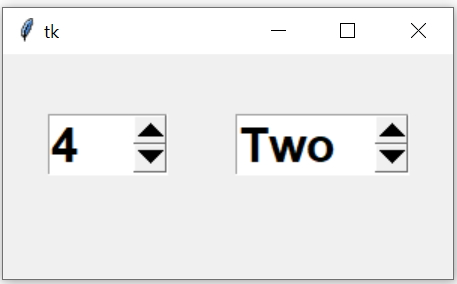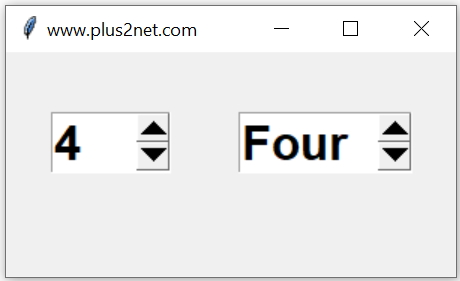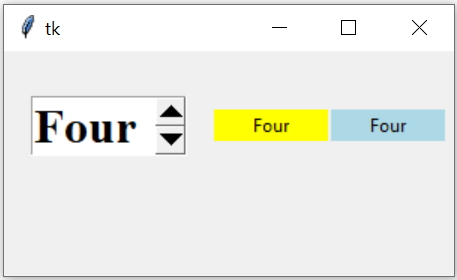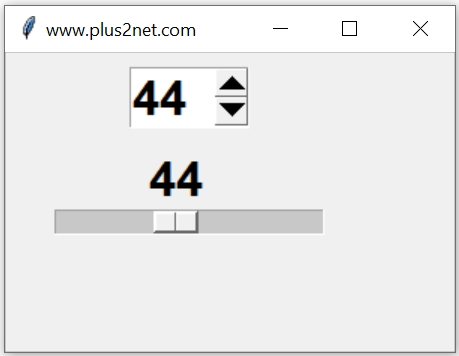# Python tkinter Spinbox

Basics of Python TkinterTkinter Spinbox to select value from given list or range and how to set and get value with options

Using Spinbox user can select value from a range of given options.
Two SpinBox widgets, one for integer and other one is for string selection by user.
``````import tkinter as tk
from tkinter import *
my_w = tk.Tk()
my_w.geometry("300x150")  # Size of the window

sb1 = Spinbox(my_w, from_= 0, to = 10,width=5)

my_list=['One', 'Two', 'Three', 'Four', 'Five']
sb2 = Spinbox(my_w,values=my_list,width=10)

my_w.mainloop()  # Keep the window open``````
User can use the arrow buttons to change the options or enter directly the value.

## How to set default value for SpinboxBy using StringVar we can set the default value of the Spinbox. Here for both the types ( number and string ) of Spinbox widgets, two different string variables ( t1 and t2 ) are used and by using set() method the default values are set.

We must set the value `t2.set('Four')` after creating the widget.
``````t1 = StringVar() # string variable
sb1 = Spinbox(my_w, from_= 0, to = 10,width=5,textvariable=t1)
t1.set(5) # set value for string variable

t2=StringVar() # string variable
my_list=['One', 'Two', 'Three', 'Four', 'Five']
sb2 = Spinbox(my_w,values=my_list,width=10,textvariable=t2)
t2.set('Four')#set value for string vaiable``````

## Reading the value of Spinbox with on change of selectionWe used two Labels here. The first Label ( l1 ) is connected to the Spinbox ( sb2 ) through StringVar t2. So once the selection of Spinbox is changed the value of StringVar t2 is reflected in Label ( l1).

The second Label ( l2 ) gets its value by this line `l2.config(text=sb2.get())` inside the function desp(). This function desp() is triggered through the command attribute of Spinbox ( `command=desp` )
``````import tkinter as tk
from tkinter import *
my_w = tk.Tk()
my_w.geometry("300x150")

def desp():
l2.config(text=sb2.get()) # 2nd Label value
print(sb2.get()) # output at console
print(t2.get())  # output at console

font1=('Times',24,'bold')
t2 = StringVar() # connected to 1st Label and Spinbox
my_tuple=['One', 'Two', 'Three', 'Four', 'Five']
sb2 = Spinbox(my_w,values=my_tuple,font=font1,width=5,
textvariable=t2, command=desp)

l1 = tk.Label(my_w,  textvariable=t2, width=10,bg='yellow' )
l1.grid(row=1,column=3)

l2 = tk.Label(my_w,  width=10 ,text='data here',bg='lightblue')
my_w.mainloop()  # Keep the window open``````

## Enable disable Spinbox

To disable we can use option state or use config().
``sb1 = Spinbox(my_w, from_= 0, to = 10, state='disabled')``
or using config()
``sb1.config(state='disabled')``
We can restore by using `state='normal'`
``sb1.config(state='normal')``
The third value of state is readonly. We can't use insert() method when `state='readonly'`
``sb1.config(state='readonly')``

## Methods

`delete(first, last=None)`: Delete one ore more char from the element.
`get`: Returns the Spinbox string ( Check above examples)
`insert(index,string)`: Place the string at Index position.
`index(index)`: Returns numerical Index.
`identify(x,y)`:Returns the name of the widget at position x, y
`invoke`:Causes the specified element to be invoked

## Using delete and insert methods

We will remove four and place FOUR as element.
``````t2 = StringVar()
my_list=['One', 'Two', 'Three', 'Four', 'Five']
sb2 = Spinbox(my_w,values=my_list,  textvariable=t2, width=10)
t2.set('Four')
sb2.delete(0,'end') # replace from 0 position till end
sb2.insert(0,'FOUR') # add string from 0 position``````
Above codes will not update any option when `state='readonly'` or `state='disabled'`

## Adding new options / values

When we used from_ and to to add a range, we can change these values by using config()
``sb1.config(from_=40,to=50) # starting from 40 to 50``
When we are using values option we can change by using list insert()
``````my_list=['One','Two','Three','Four','Five']
sb2=tk.Spinbox(my_w,values=my_list,width=10,textvariable=t2)
sb2.grid(row=1,column=2)
my_list.insert(5,'Six') # added option six at index position 5
sb2.config(values=my_list)``````

## Inter connecting Spinbox and Scale widgets

We can add one horizontal scrollbar by using one Scale widget. Here both are connected so changes in any one will reflect in both.
We used one common StringVar sv here.``````import tkinter as tk
from tkinter import *
my_w = tk.Tk()
my_w.geometry("300x200")
my_w.title("www.plus2net.com")

font1=('Arial',24,'bold')
sv = StringVar() #string variable
sb = Spinbox(my_w,textvariable=sv,font=font1,
width=3,from_=0,to=100)

sc = Scale(my_w, from_=0, to=100, font=font1,
orient=HORIZONTAL,variable=sv,length=180)

my_w.mainloop()  # Keep the window open``````

## Options

 `activebackground` Color of the slider when Mouse is over it. `bg` Color of the slider and arrowheads when Mouse is NOT over it. `bd` Border width of the widget `command` When state is changed call the function. ( Example above ) `bd` Border width of the widget `cursor` Shape of the cursor while moving over the Spinbox ( List of cursor shapes ) `disabledbackground` Background color when it is disabled``````sb1 = Spinbox(my_w, from_= 0, to = 10, disabledbackground='yellow',state='disabled')`````` `disabledforeground` Foreground color when it is disabled``````sb1 = Spinbox(my_w, from_= 0, to = 10, disabledforeground='blue',state='disabled')`````` `font` Font family size style ``````my_font=('Times',18,'normal') sb1 = Spinbox(my_w, from_= 0, to = 10, font=my_font)`````` `fg` Foreground colour ``sb1 = Spinbox(my_w, from_= 0, to = 10,fg='blue')`` `format` Format the display, showing decimal place or starting with zero etc. ``sb1 = Spinbox(my_w, from_= 00, to = 60,format='%02.02f')`` `from_` Start value of Spinbox, check examples above. `increment` Step value of change when we use the arrow buttons. `justify` Alignment of text inside. ( left , center, right ) ``sb1 = Spinbox(my_w, from_= 0, to = 60,justify='right')`` `relief` Border style, ( raised, sunken,flat,ridge,solid,groove ) Check the Button for sample images``sb1 = Spinbox(my_w, from_= 0, to = 60,relief='solid')`` `state` state of Spinbox , ( disabled, normal, readonly) Check the examples above ``sb1 = Spinbox(my_w, from_= 0, to = 60,state='disabled')`` `validate` When to Validate the input ( none,focus,focusin,focusout,key,all) ``sb1 = Spinbox(my_w, from_= 0, to = 60,validate='key')`` `validatecommand` Callback function to execute validation ``````sb1 = Spinbox(my_w, from_= 0, to = 10, validate='key',validatecommand=(my_valid,'%s'))`````` More on input Validation `to` End value of Spinbox, check examples above. `repeatdelay` Delay in changing value when you press the Up or down arrow and holding it ( for first time ) in milliseconds``sb1 = Spinbox(my_w,from_=0,to=100,repeatdelay=4000)``What is the difference between repeatdelay and repeatinterval ? `repeatinterval` Delay in changing value in each step when you press the Up or down arrow and holding it in milliseconds``sb1 = Spinbox(my_w,from_=0,to =100,repeatinterval=3000)``Why in this code the first change is not matching with repeatinterval of 3 sec ? Try to add repeatdelay and see the difference `xscrollcommand` Used with set method of scrollbar `wrap` Boolean, default value is False. If it is set to True then value starts ( repeat ) from lower limit ( from_) after the maximum value ( to ) is reached. ``sb1 = Spinbox(my_w, from_= 0, to = 10,wrap=True)`` `width` Control the width of the spinbox ( examples above) `vcmd` See validatecommand above `values` The options we can add, see examples above (sb2) `textvariable` The variable to connect to the widget, check the examples above where we used t2 the StrVariable to sb2

Displaying incremental data based on timer with step value & upper limit in Entry widget of Tkinter

## Projects using Spinbox

1. Display two Spinboxes showing numbers and on click of a button display the sum of the selected numbers.
2. Collect all student names from MySQL table and display them using Spinbox. On submit of a button display full details of the selected record ( student ).

Subscribe to our YouTube Channel here

## Subscribe

* indicates required
Subscribe to plus2netplus2net.com